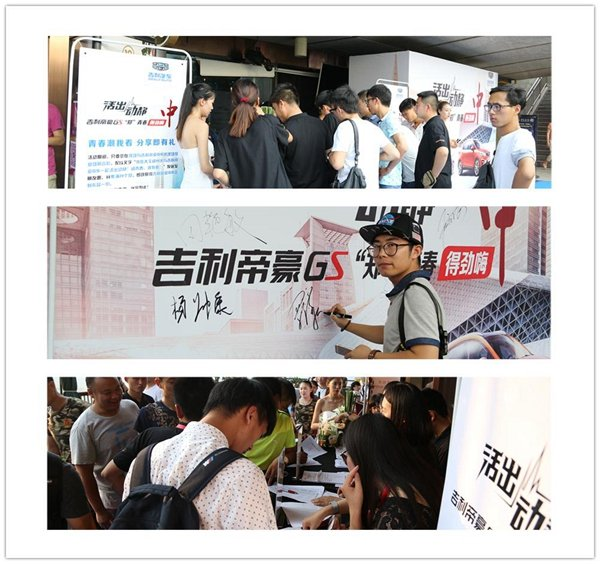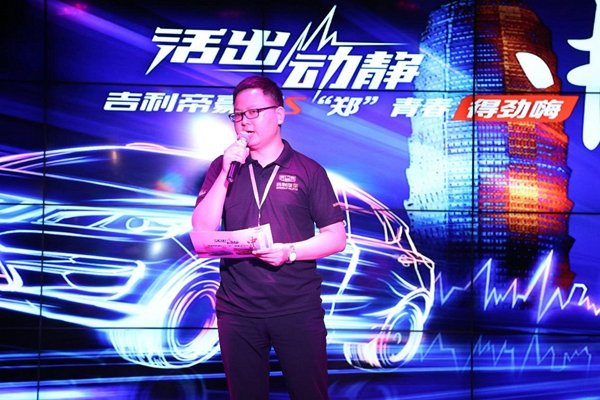## 吉利帝豪GS动静派对“郑”青春嗨翻全城

2016年07月25日 17:02 来源：www.chextx.com 超过：次关注

伴着燃情的盛夏，7月24日“活出动静”吉利帝豪GS郑州动静派对在郑州千玺广场18港式餐厅酒吧火热开启；这是一场无关乎年龄，只关乎心态的聚会，吉利帝豪GS与古老而又年轻的郑州邂逅迸发动静，为青春正言；新颖、动感、活力的动静狂欢之夜吸引了超过400位客户、嘉宾以及媒体的到场，与吉利帝豪GS为伴，誓把青春再拉长。在活动开始前，吉利帝豪GS的巡游车队浩浩荡荡的行驶在这个古老而年轻的城市时；以此感受郑州的胸怀和温柔。虽然郑州是中原文化的根基之地，但这的人却是带着青春的梦想与年轻的态度生活；吉利帝豪GS那独特的城市跨界SUV英姿、精致具匠心的内饰在动静路上渲染着青春，车队所到之处便是一道亮丽的风景线；360度安全科技全方位周全保护，郑州的喧闹繁华轻松应对；作为吉利汽车3.0精品时代首款城市跨界SUV，吉利帝豪GS综合了运动气息，为青春正言。不管动静大小，跨出那一步便已是人生赢家。

五彩斑斓的灯光与音乐欢迎着每一位到场的嘉宾，集时尚、科技、动感为一体的动静派对成为诠释85后时尚新知人群对于活出动静的最好表达。“高颜值”的吉利帝豪GS更是惹出不小的动静，人们纷纷打量着、赞许这宛如艺术般的匠心之作。当现场嘉宾还沉醉在吉利帝豪GS的魅力中时，热辣的模特走秀在这动静之间瞬间点燃现场气氛，而乐队那一首首脍炙人口的歌曲惹来全场大合唱。余音还在，当现场嘉宾朋友看到自己儿时钟爱的经典游戏时，都争先恐后的报名参加，顿时现场一片欢乐动静之声。

不仅如此，活动还邀请到吉利帝豪GS首批新晋车主，为其举办隆重的交车仪式，在众人的祝福与掌声中接过新车钥匙，让他们感受到吉利这个大家庭的满满诚意与暖暖情怀。而本次动静派对最受瞩目的现场抽奖环节更是将现场的活力迸发到极致，获奖嘉宾均获得吉利帝豪GS所带来的重磅大奖。

随着大奖的名花有主，本次动静派对也接近尾声；在这场动静之间的相遇，“活出动静”的理念也得到年轻消费群体的广泛关注与认同。感受着吉利帝豪GS带来的激情。体验着青春不老的活力。作为与青年群体沟通的情感纽带，吉利帝豪GS以新颖的方式与用户积极互动，用极致的产品、极致的价值打动用户，让消费者感受到吉利帝豪GS不同寻常的“动静”。

#### 相关文章

﻿
• 快速找车
• 选择品牌
• 选择品牌
• A  奥迪
• A  阿斯顿·马丁
• A  阿尔法·罗密欧
• B  宝沃
• B  布加迪
• B  巴博斯
• B  保时捷
• B  宾利
• B  奔驰
• B  宝马
• B  本田
• B  别克
• B  标致
• B  比亚迪
• B  宝骏
• B  北汽制造
• B  北汽新能源
• B  北汽幻速
• B  北汽威旺
• B  北京汽车
• B  奔腾
• B  北汽绅宝
• B  北汽昌河
• C  长安欧尚
• C  长安
• C  长安凯程
• C  长城
• D  大众
• D  道奇
• D  DS
• D  东南
• D  东风风神
• D  东风风行
• D  东风小康
• D  东风风度
• D  东风
• F  福特
• F  丰田
• F  菲亚特
• F  法拉利
• F  福田
• F  福迪
• F  福汽启腾
• G  观致
• G  广汽传祺
• G  广汽吉奥
• G  GMC
• H  红旗
• H  汉腾汽车
• H  哈弗
• H  哈飞
• H  海格
• H  海马
• H  华颂
• H  黄海
• H  华泰
• H  恒天
• J  几何汽车
• J  捷达
• J  吉利汽车
• J  捷豹
• J  Jeep
• J  江淮
• J  江铃
• J  金杯
• J  九龙
• J  金旅
• K  凯翼
• K  凯迪拉克
• K  克莱斯勒
• K  科尼塞克
• K  卡威
• K  开瑞
• L  路虎
• L  林肯
• L  劳斯莱斯
• L  兰博基尼
• L  雷克萨斯
• L  铃木
• L  领克
• L  雷诺
• L  理念
• L  力帆
• L  莲花汽车
• L  猎豹
• L  路特斯
• L  陆风
• M  马自达
• M  MG
• M  MINI
• M  玛莎拉蒂
• M  摩根
• M  迈凯轮
• N  纳智捷
• O  欧拉
• O  欧宝
• O  讴歌
• O  欧朗
• Q  奇瑞
• Q  起亚
• Q  启辰
• R  日产
• R  荣威
• R  瑞麒
• S  SERES赛力斯
• S  三菱
• S  斯威汽车
• S  萨博
• S  smart
• S  斯柯达
• S  斯巴鲁
• S  思铭
• S  双龙
• S  上汽大通
• S  双环
• T  特斯拉
• T  腾势
• W  蔚来
• W  沃尔沃
• W  WEY
• W  五菱汽车
• W  五十铃
• W  威兹曼
• W  威麟
• X  现代
• X  雪佛兰
• X  星途
• X  雪铁龙
• X  小鹏汽车
• X  西雅特
• Y  一汽
• Y  英菲尼迪
• Y  英致
• Y  依维柯
• Y  野马汽车
• Y  永源
• Z  众泰
• Z  中华
• Z  中兴
• Z  知豆
• 选择车系
• 选择车系
• 车型对比
• 选择品牌
• 选择品牌
• A  奥迪
• A  阿斯顿·马丁
• A  阿尔法·罗密欧
• B  宝沃
• B  布加迪
• B  巴博斯
• B  保时捷
• B  宾利
• B  奔驰
• B  宝马
• B  本田
• B  别克
• B  标致
• B  比亚迪
• B  宝骏
• B  北汽制造
• B  北汽新能源
• B  北汽幻速
• B  北汽威旺
• B  北京汽车
• B  奔腾
• B  北汽绅宝
• B  北汽昌河
• C  长安欧尚
• C  长安
• C  长安凯程
• C  长城
• D  大众
• D  道奇
• D  DS
• D  东南
• D  东风风神
• D  东风风行
• D  东风小康
• D  东风风度
• D  东风
• F  福特
• F  丰田
• F  菲亚特
• F  法拉利
• F  福田
• F  福迪
• F  福汽启腾
• G  观致
• G  广汽传祺
• G  广汽吉奥
• G  GMC
• H  红旗
• H  汉腾汽车
• H  哈弗
• H  哈飞
• H  海格
• H  海马
• H  华颂
• H  黄海
• H  华泰
• H  恒天
• J  几何汽车
• J  捷达
• J  吉利汽车
• J  捷豹
• J  Jeep
• J  江淮
• J  江铃
• J  金杯
• J  九龙
• J  金旅
• K  凯翼
• K  凯迪拉克
• K  克莱斯勒
• K  科尼塞克
• K  卡威
• K  开瑞
• L  路虎
• L  林肯
• L  劳斯莱斯
• L  兰博基尼
• L  雷克萨斯
• L  铃木
• L  领克
• L  雷诺
• L  理念
• L  力帆
• L  莲花汽车
• L  猎豹
• L  路特斯
• L  陆风
• M  马自达
• M  MG
• M  MINI
• M  玛莎拉蒂
• M  摩根
• M  迈凯轮
• N  纳智捷
• O  欧拉
• O  欧宝
• O  讴歌
• O  欧朗
• Q  奇瑞
• Q  起亚
• Q  启辰
• R  日产
• R  荣威
• R  瑞麒
• S  SERES赛力斯
• S  三菱
• S  斯威汽车
• S  萨博
• S  smart
• S  斯柯达
• S  斯巴鲁
• S  思铭
• S  双龙
• S  上汽大通
• S  双环
• T  特斯拉
• T  腾势
• W  蔚来
• W  沃尔沃
• W  WEY
• W  五菱汽车
• W  五十铃
• W  威兹曼
• W  威麟
• X  现代
• X  雪佛兰
• X  星途
• X  雪铁龙
• X  小鹏汽车
• X  西雅特
• Y  一汽
• Y  英菲尼迪
• Y  英致
• Y  依维柯
• Y  野马汽车
• Y  永源
• Z  众泰
• Z  中华
• Z  中兴
• Z  知豆
• 选择车系
• 选择车系
• 选择车型
• 选择车型
• 意见反馈